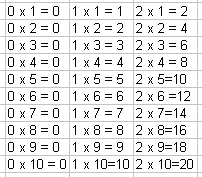Name: ___________________Date:___________________

 Email us to get an instant 20% discount on highly effective K-12 Math & English kwizNET Programs!

### Grade 4 - Mathematics3.2 Multiplication Facts 0-5Directions: Review the time table and answer the following questions. Also write ten examples of your own and multiplication facts for 0,1,2,3,4,5 on a sheet of paper.
 Q 1: 5 x 4=20911 Q 2: 5 x 3 =8152 Q 3: 4 x 4=81610 Q 4: 3 x 6 =3918 Q 5: 5 x 7=303545 Q 6: 10 x 1 =01011 Q 7: 9 x 11 =209990 Q 8: 10 x 1 =11100 Question 9: This question is available to subscribers only! Question 10: This question is available to subscribers only!